1624438800

# What are Decision Tree Algorithms? 🌳

This article will cover one of the most advanced algorithms and most widely used in analytical applications. This is an extensive subject, as we have several algorithms and various techniques for working with decision trees.

On the other hand, these algorithms are among the most powerful in Machine Learning and are easy to interpret. So, let’s start by defining what decision trees are and their representation through machine learning algorithms.

For decision tree learning models, we will study some algorithms with C4.5, C5.0, CART, and ID3. In addition, there are some specialized types of decision trees, and we will learn this following the chapter.

The main specialization of decision trees is RandomForest, which is nothing more than a collection of decision trees. We can use RandomForest for attribute selection, i.e., we can use decision trees for Machine Learning models themselves and apply feature selection techniques to prepare our dataset for other machine learning algorithms.

Finally, we will create models, make predictions, study the parameters and pre-processing details of decision trees, and interpret the results of predictive models.

When creating decision trees, we can have trees with lots of branches and leaves, and at some point, we will have to stop the construction of the tree or make adjustments to reduce the number of decision points in the predictive model.

## Decision Trees

This machine learning technique is easy to interpret; that is, we can quickly solve the result of a Decision Tree model, RandomForest, or even an Ensemble method, unlike other techniques such as Artificial Neural Networks or Deep Learning challenging to interpret the result.

Decision Trees are known as one of the most powerful and widely used machine learning modeling techniques. Decision Trees can naturally induce rules that can be used for data classification or to make predictions.

A decision tree is a decision support tool. Graphically presents the shape of an upside-down tree, where the root is at the top, and the leaves are at the bottom.

#big-data #machine-learning #programming #artificial-intelligence #algorithms #decision tree

## Buddha Community1596428520

## Decision Tree Algorithm..

Decision tree is one of the popular machine learning algorithms which is the stepping stone to understand the ensemble techniques using trees.

Also, Decision Tree algorithm is a hot topic in many of the interviews which are conducted related to data science field.

Understanding Decision Tree…

Decision Tree is more of a kind of Management tool which is used by many professionals to take decisions regarding the resource costs, decision to be made on the basis of filters applied.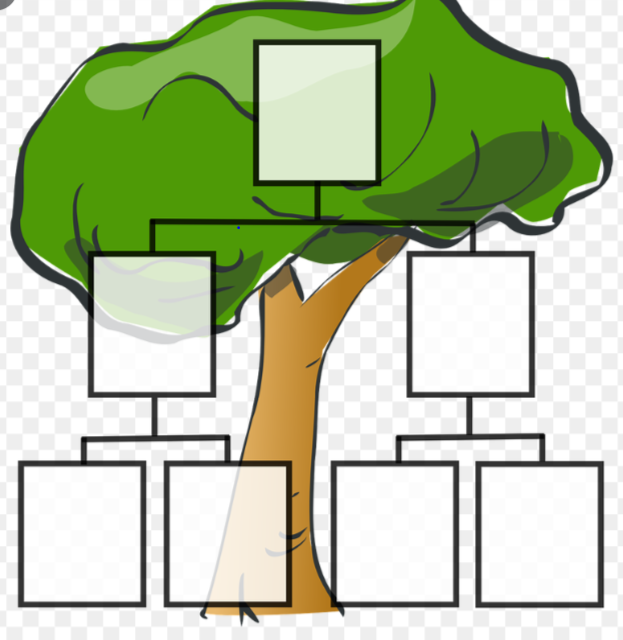The best part of a Decision Tree is that it is a non-parametric tool, which means that there are no underlying assumptions about the distribution of the errors or the data. It basically means that the model is constructed based on the observed data.

They are adaptable at solving any kind of problem at hand (classification or regression). Decision Tree algorithms are referred to as CART (Classification and Regression Trees).

Common terms used with Decision trees:

1. Root Node: It represents entire population or sample and this further gets divided into two or more homogeneous sets.
2. Splitting: It is a process of dividing a node into two or more sub-nodes.
3. Decision Node: When a sub-node splits into further sub-nodes, then it is called decision node.
4. Leaf/ Terminal Node: Nodes do not split is called Leaf or Terminal node.
5. **Max_Depth: **The complete journey of a tree from root till the leaf nodes.
6. Branch / Sub-Tree: A sub section of entire tree is called branch or sub-tree.
7. Parent and Child Node: A node, which is divided into sub-nodes is called parent node of sub-nodes whereas sub-nodes are the child of parent node.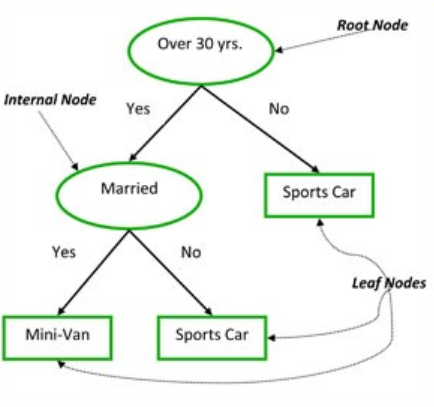classic example to demonstrate a Decision Tree

How a Decision Tree works!

1. First, we will iterate through all possible splits, calculate the purity of each split and pick the split.
2. The purity is compared across all labels and best is chosen. This makes the root node as best predictor.
3. This algorithm is recursive in nature as the groups formed can be sub-divided and the process is repeated till the tree is fully grown.

Main Decision Areas:

1. Determine the best split:

The node with homogeneous class distribution are preferred.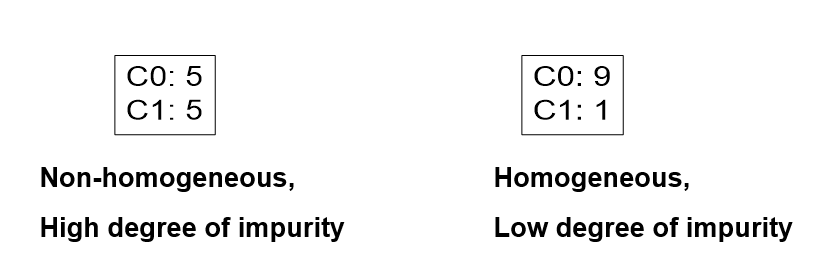2. Measures of Node Impurity: Below are the measures of the impurity

(a). Gini Index

(b). Entropy

Understanding each terminologies with the example:

Let us take a dataset- weather, below is the snapshot of the header of the data: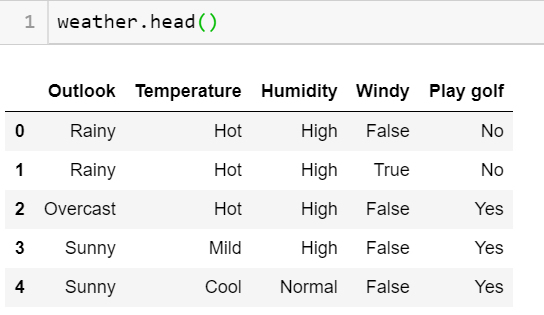Now according to the algorithm written above and the decision points to be considered, we need the feature having maximum information split possible.

Note: At the root node, the impurity level will be maximum with negligible information gain. As we go down the tree, the Entropy reduces with maximizing the Information gain.Therefore, we choose a feature with maximum gain achieved.

#data-science #machine-learning #decision-tree #algorithms #algorithms1596286260

## Decision Tree -Classification

Decision Tree is one of the most widely used machine learning algorithm. It is a supervised learning algorithm that can perform both classification and regression operations.

As the name suggest, it uses a tree like structure to make decisions on the given dataset. Each internal node of the tree represent a “decision” taken by the model based on any of our attributes. From this decision, we can seperate classes or predict values.

Let’s look at both classification and regression operations one by one.

# Classification

In Classification, each leaf node of our decision tree represents a **class **based on the decisions we make on attributes at internal nodes.

To understand it more properly let us look at an example. I have used the Iris Flower Dataset from sklearn library. You can refer the complete code on Github — Here.

Image for post

A node’s samples attribute counts how many training instances it applies to. For example, 100 training instances have a petal width ≤ 2.45 cm .

A node’s value attribute tells you how many training instances of each class this node applies to. For example, the bottom-right node applies to 0 Iris-Setosa, 0 Iris- Versicolor, and 43 Iris-Virginica.

And a node’s gini attribute measures its impurity: a node is “pure” (gini=0) if all training instances it applies to belong to the same class. For example, since the depth-1 left node applies only to Iris-Setosa training instances, it is pure and its gini score is 0.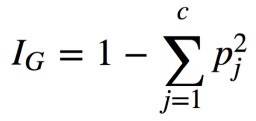Gini Impurity Formula

where, pⱼ is the ratio of instances of class j among all training instances at that node.

Based on the decisions made at each internal node, we can sketch decision boundaries to visualize the model.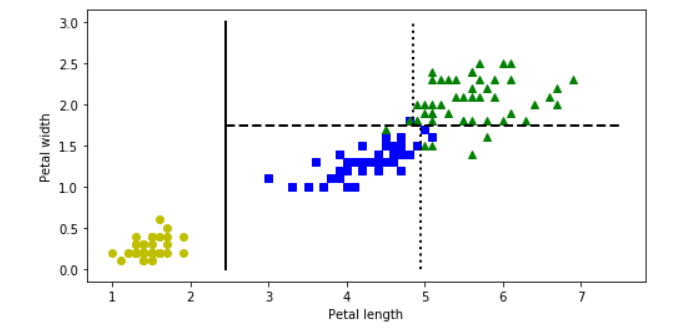But how do we find these boundaries ?

We use Classification And Regression Tree (CART) to find these boundaries.

CART is a simple algorithm that finds an attribute _k _and a threshold _t_ₖat which we get a purest subset. Purest subset means that either of the subsets contain maximum proportion of one particular class. For example, left node at depth-2 has maximum proportion of Iris-Versicolor class i.e 49 of 54. In the _CART cost function, _we split the training set in such a way that we get minimum gini impurity.The CART cost function is given as: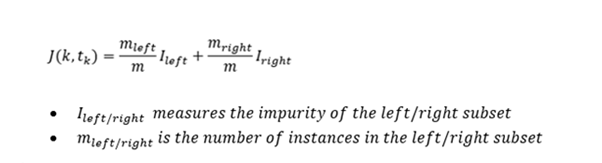After successfully splitting the dataset into two, we repeat the process on either sides of the tree.

We can directly implement Decision tree with the help of Scikit learn library. It has a class called DecisionTreeClassifier which trains the model for us directly and we can adjust the hyperparameters as per our requirements.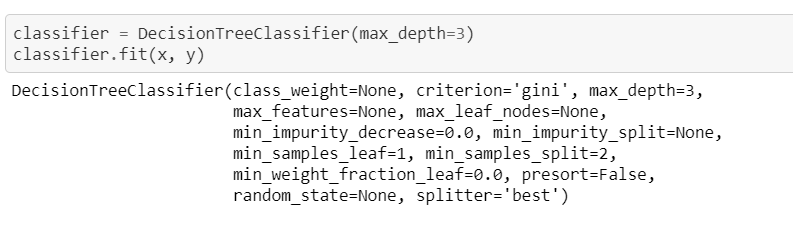#machine-learning #decision-tree #decision-tree-classifier #decision-tree-regressor #deep learning1624438800

## What are Decision Tree Algorithms? 🌳

This article will cover one of the most advanced algorithms and most widely used in analytical applications. This is an extensive subject, as we have several algorithms and various techniques for working with decision trees.

On the other hand, these algorithms are among the most powerful in Machine Learning and are easy to interpret. So, let’s start by defining what decision trees are and their representation through machine learning algorithms.

For decision tree learning models, we will study some algorithms with C4.5, C5.0, CART, and ID3. In addition, there are some specialized types of decision trees, and we will learn this following the chapter.

The main specialization of decision trees is RandomForest, which is nothing more than a collection of decision trees. We can use RandomForest for attribute selection, i.e., we can use decision trees for Machine Learning models themselves and apply feature selection techniques to prepare our dataset for other machine learning algorithms.

Finally, we will create models, make predictions, study the parameters and pre-processing details of decision trees, and interpret the results of predictive models.

When creating decision trees, we can have trees with lots of branches and leaves, and at some point, we will have to stop the construction of the tree or make adjustments to reduce the number of decision points in the predictive model.

## Decision Trees

This machine learning technique is easy to interpret; that is, we can quickly solve the result of a Decision Tree model, RandomForest, or even an Ensemble method, unlike other techniques such as Artificial Neural Networks or Deep Learning challenging to interpret the result.

Decision Trees are known as one of the most powerful and widely used machine learning modeling techniques. Decision Trees can naturally induce rules that can be used for data classification or to make predictions.

A decision tree is a decision support tool. Graphically presents the shape of an upside-down tree, where the root is at the top, and the leaves are at the bottom.

#big-data #machine-learning #programming #artificial-intelligence #algorithms #decision tree1592847556

## Binary Decision Trees

Binary Decision Trees. Binary Decision Trees
Binary decision trees is a supervised machine-learning technique operates by subjecting attributes to a series of binary (yes/no) decisions. Each decision leads to one of two possibilities. Each decision leads to another decision or it leads to prediction.

#decision-tree-regressor #decision-tree #artificial-intelligence #mls #machine-learning #programming1602997200

## Decision Tree Intuition

Decision Trees are easy & Simple to implement & interpreted. Decision Tree is a diagram (flow) that is used to predict the course of action or a probability. Each branch of the decision tree represents an outcome or decision or a reaction. Decision Trees can be implemented in a variety of situations from personal to complex situations. The sequence of steps will give a better understanding easily.

In Programming, we regularly use If-else conditions, even the Decision Tree working process is similar to an If-else condition.

## Let’s see how a decision Tree Looks like

The below tree shows a simple implementation of different nodes in the decision tree.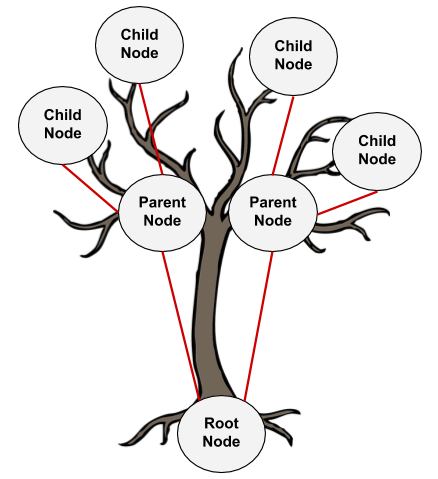1. **Root Node: **Root Node is a top node with the base feature.
2. **Parent Node: **Nodes that get their origin from a root node or this can be represented as a decision node where the decision of Yes/No or True/False or prediction turn happens.
3. **Child Node: **these nodes get their origin from a parent node. If the decision made from a parent node is not satisfactory then these nodes will be created. Until we arrive at the final node where we have pure domination in a class (Yes/No) means that until we arrive at the leaf node.
4. **Leaf Node: **Can also be called a terminal Node or a final decision node where we will conclude.

#gini-index #entropy #decision-tree #decision-tree-classifier #machine-learning